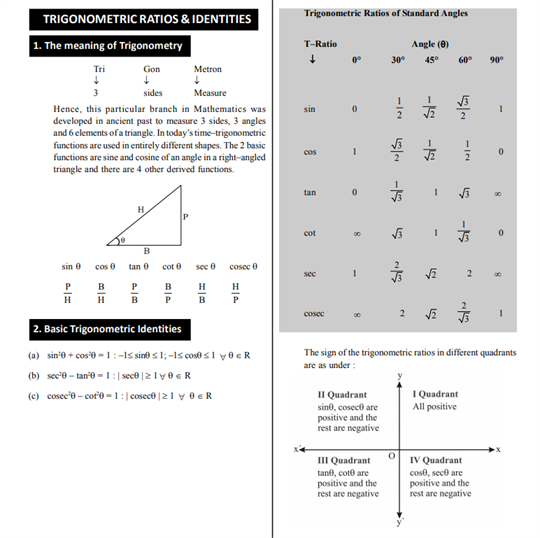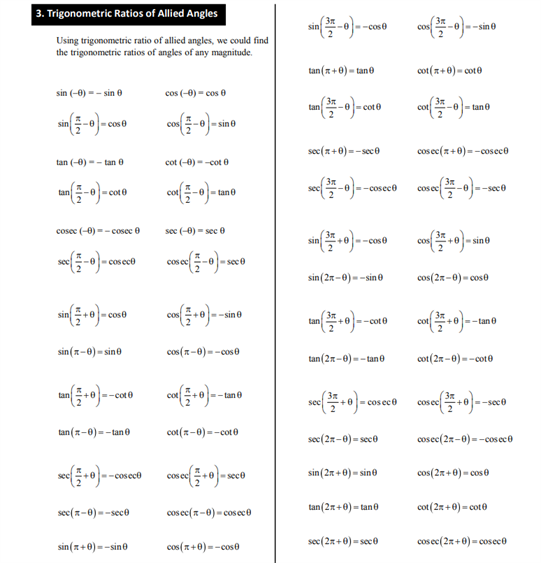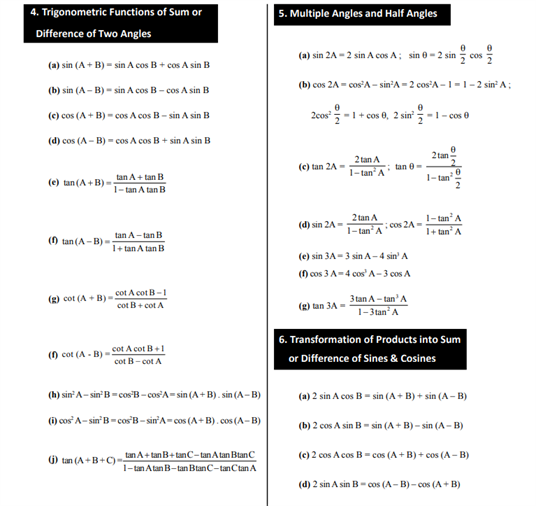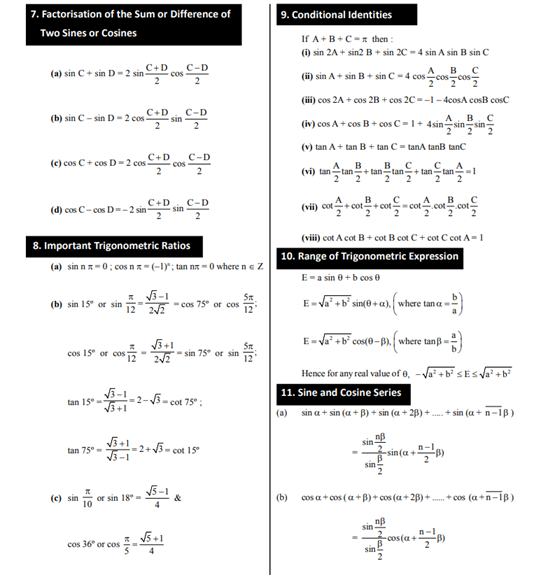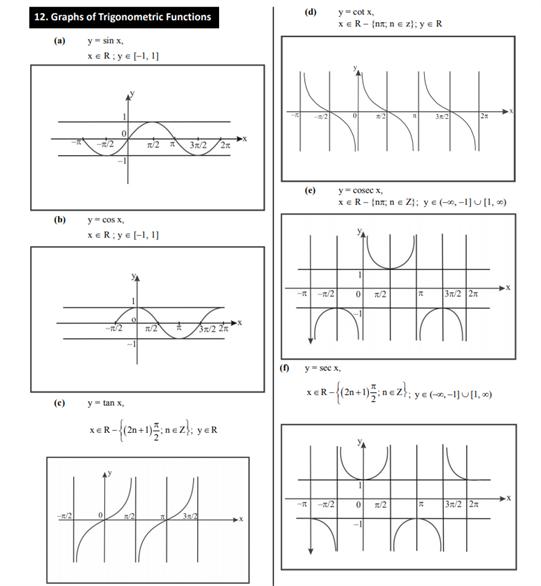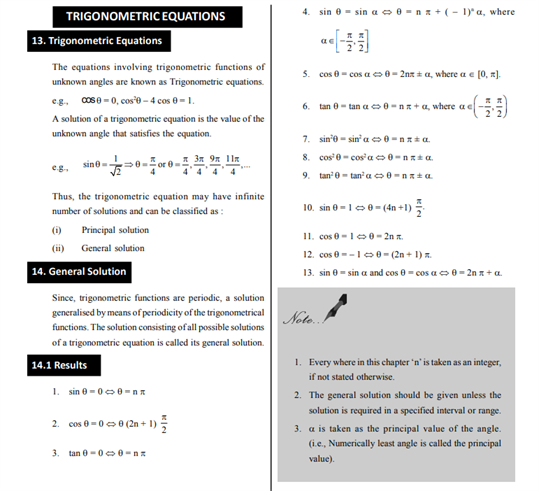# Revision Notes For CBSE Class 11 Maths Chapter 3 Trigonometric Functions

Class 11 maths revision notes Chapter 3 Trigonometric Functions has a detailed explanation of all the important concepts related to this chapter. These notes are arranged systematically for student’s ease and students are recommended to refer these notes whenever feel necessary. The content is made interactive in these notes so that students can prepare effectively from it. We shall find the definition of trigonometric function below:

• Trigonometric functions are elementary functions, the argument of which is an angle. Trigonometric functions describe the relation between the sides and angles of a right triangle. Applications of trigonometric functions are extremely diverse. For example, any periodic processes can be represented as a sum of trigonometric functions (Fourier series). These functions often appear in the solution of differential equations and functional equations.
• The trigonometric functions include the following functions: sine, cosine, tangent, cotangent, secant, and cosecant. For each of these functions, there is an inverse trigonometric function.

With the help of this note, viewers will understand the basic trigonometric identities, trigonometric ratios of allied angles, the difference of two angles, conditional identities and also witness graphs of trigonometric functions which is properly arranged for easy preparation. These notes are must to read material when preparing for exams.

Find out the class 11 maths revision notes Chapter 3 Trigonometric Functions below.